# Signal Classification Using Wavelet-Based Features and Support Vector Machines

This example shows how to classify human electrocardiogram (ECG) signals using wavelet-based feature extraction and a support vector machine (SVM) classifier. The problem of signal classification is simplified by transforming the raw ECG signals into a much smaller set of features that serve in aggregate to differentiate different classes. You must have Wavelet Toolbox™, Signal Processing Toolbox™, and Statistics and Machine Learning Toolbox™ to run this example. The data used in this example are publicly available from PhysioNet.

### Data Description

This example uses ECG data obtained from three groups, or classes, of people: persons with cardiac arrhythmia, persons with congestive heart failure, and persons with normal sinus rhythms. The example uses 162 ECG recordings from three PhysioNet databases: MIT-BIH Arrhythmia Database , MIT-BIH Normal Sinus Rhythm Database , and The BIDMC Congestive Heart Failure Database . In total, there are 96 recordings from persons with arrhythmia, 30 recordings from persons with congestive heart failure, and 36 recordings from persons with normal sinus rhythms. The goal is to train a classifier to distinguish between arrhythmia (ARR), congestive heart failure (CHF), and normal sinus rhythm (NSR).

The first step is to download the data from the GitHub repository. To download the data, click Clone or download and select Download ZIP. Save the file physionet_ECG_data-master.zip in a folder where you have write permission. The instructions for this example assume you have downloaded the file to your temporary directory, (tempdir in MATLAB). Modify the subsequent instructions for unzipping and loading the data if you choose to download the data in folder different from tempdir. If you are familiar with Git, you can download the latest version of the tools (git) and obtain the data from a system command prompt using git clone https://github.com/mathworks/physionet_ECG_data/ .

The file physionet_ECG_data-master.zip contains

• ECGData.zip

and ECGData.zip contains

• ECGData.mat

• Modified_physionet_data.txt

ECGData.mat holds the data used in this example. The .txt file, Modified_physionet_data.txt, is required by PhysioNet's copying policy and provides the source attributions for the data as well as a description of the pre-processing steps applied to each ECG recording.

If you followed the download instructions in the previous section, enter the following commands to unzip the two archive files.

unzip(fullfile(tempdir,'physionet_ECG_data-master.zip'),tempdir) unzip(fullfile(tempdir,'physionet_ECG_data-master','ECGData.zip'),... fullfile(tempdir,'ECGData')) 

After you unzip the ECGData.zip file, load the data into MATLAB.

load(fullfile(tempdir,'ECGData','ECGData.mat')) 

ECGData is a structure array with two fields: Data and Labels. Data is a 162-by-65536 matrix where each row is an ECG recording sampled at 128 hertz. Labels is a 162-by-1 cell array of diagnostic labels, one for each row of Data. The three diagnostic categories are: 'ARR' (arrhythmia), 'CHF' (congestive heart failure), and 'NSR' (normal sinus rhythm).

### Create Training and Test Data

Randomly split the data into two sets - training and test data sets. The helper function helperRandomSplit performs the random split. helperRandomSplit accepts the desired split percentage for the training data and ECGData. The helperRandomSplit function outputs two data sets along with a set of labels for each. Each row of trainData and testData is an ECG signal. Each element of trainLabels and testLabels contains the class label for the corresponding row of the data matrices. In this example, we randomly assign 70% percent of the data in each class to the training set. The remaining 30% is held out for testing (prediction) and are assigned to the test set.

percent_train = 70; [trainData,testData,trainLabels,testLabels] = ... helperRandomSplit(percent_train,ECGData); 

There are 113 records in the trainData set and 49 records in testData. By design the training data contains 69.75% (113/162) of the data. Recall that the ARR class represents 59.26% of the data (96/162), the CHF class represents 18.52% (30/162), and the NSR class represents 22.22% (36/162). Examine the percentage of each class in the training and test sets. The percentages in each are consistent with the overall class percentages in the data set.

Ctrain = countcats(categorical(trainLabels))./numel(trainLabels).*100 Ctest = countcats(categorical(testLabels))./numel(testLabels).*100 
Ctrain = 59.2920 18.5841 22.1239 Ctest = 59.1837 18.3673 22.4490 

### Plot Samples

Plot the first few thousand samples of four randomly selected records from ECGData. The helper function helperPlotRandomRecords does this. helperPlotRandomRecords accepts ECGData and a random seed as input. The initial seed is set at 14 so that at least one record from each class is plotted. You can execute helperPlotRandomRecords with ECGData as the only input argument as many times as you wish to get a sense of the variety of ECG waveforms associated with each class. You can find the source code for this helper function in the Supporting Functions section at the end of this example.

helperPlotRandomRecords(ECGData,14)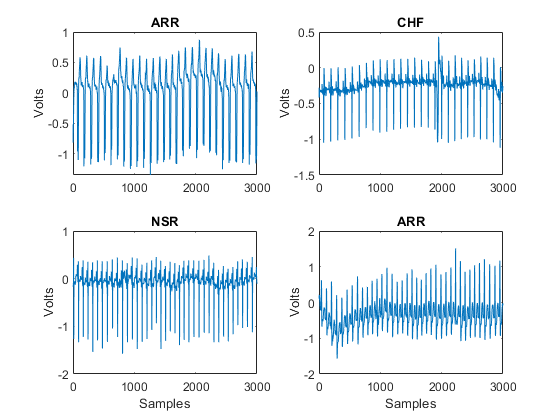### Feature Extraction

Extract the features used in the signal classification for each signal. This example uses the following features extracted on 8 blocks of each signal approximately one minute in duration (8192 samples):

• Autoregressive model (AR) coefficients of order 4 .

• Shannon entropy (SE) values for the maximal overlap discrete wavelet packet transform (MODPWT) at level 4 .

• Multifractal wavelet leader estimates of the second cumulant of the scaling exponents and the range of Holder exponents, or singularity spectrum .

Additionally, multiscale wavelet variance estimates are extracted for each signal over the entire data length . An unbiased estimate of the wavelet variance is used. This requires that only levels with at least one wavelet coefficient unaffected by boundary conditions are used in the variance estimates. For a signal length of 2^16 (65,536) and the 'db2' wavelet this results in 14 levels.

These features were selected based on published research demonstrating their effectiveness in classifying ECG waveforms. This is not intended to be an exhaustive or optimized list of features.

The AR coefficients for each window are estimated using the Burg method, arburg. In , the authors used model order selection methods to determine that an AR(4) model provided the best fit for ECG waveforms in a similar classification problem. In , an information theoretic measure, the Shannon entropy, was computed on the terminal nodes of a wavelet packet tree and used with a random forest classifier. Here we use the nondecimated wavelet packet transform, modwpt, down to level 4.

The definition of the Shannon entropy for the undecimated wavelet packet transform following  is given by:whereis the number of the corresponding coefficients in the j-th node andare the normalized squares of the wavelet packet coefficients in the j-th terminal node.

Two fractal measures estimated by wavelet methods are used as features. Following , we use the width of the singularity spectrum obtained from dwtleader as a measure of the multifractal nature of the ECG signal. We also use the second cumulant of the scaling exponents. The scaling exponents are scale-based exponents describing power-law behavior in the signal at different resolutions. The second cumulant broadly represents the departure of the scaling exponents from linearity.

The wavelet variance for the entire signal is obtained using modwtvar. Wavelet variance measures variability in a signal by scale, or equivalently variability in a signal over octave-band frequency intervals.

In total there are 190 features: 32 AR features (4 coefficients per block), 128 Shannon entropy values (16 values per block), 16 fractal estimates (2 per block), and 14 wavelet variance estimates.

The helperExtractFeatures function computes these features and concatenates them into a feature vector for each signal. You can find the source code for this helper function in the Supporting Functions section at the end of this example.

timeWindow = 8192; ARorder = 4; MODWPTlevel = 4; [trainFeatures,testFeatures,featureindices] = ... helperExtractFeatures(trainData,testData,timeWindow,ARorder,MODWPTlevel); 

trainFeatures and testFeatures are 113-by-190 and 49-by-190 matrices, respectively. Each row of these matrices is a feature vector for the corresponding ECG data in trainData and testData, respectively. In creating feature vectors, the data is reduced from 65536 samples to 190 element vectors. This is a significant reduction in data, but the goal is not just a reduction in data. The goal is to reduce the data to a much smaller set of features which captures the difference between the classes so that a classifier can accurately separate the signals. The indices for the features, which make up both trainFeatures and testFeatures are contained in the structure array, featureindices. You can use these indices to explore features by group. As an example, examine the range of Holder exponents in the singularity spectra for the first time window. Plot the data for the entire data set.

allFeatures = [trainFeatures;testFeatures]; allLabels = [trainLabels;testLabels]; figure boxplot(allFeatures(:,featureindices.HRfeatures(1)),allLabels,'notch','on') ylabel('Holder Exponent Range') title('Range of Singularity Spectrum by Group (First Time Window)') grid on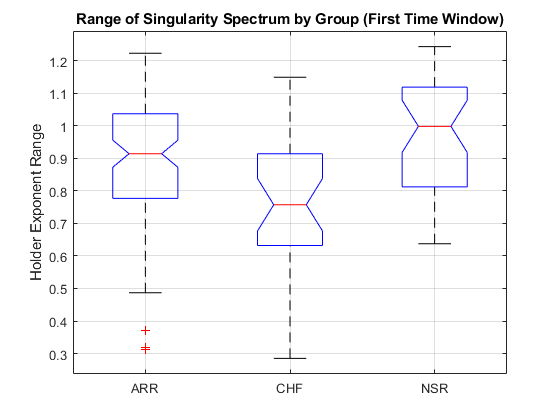You can perform a one-way analysis of variance on this feature and confirm what appears in the boxplot, namely that the ARR and NSR groups have a significantly larger range than the CHF group.

[p,anovatab,st] = anova1(allFeatures(:,featureindices.HRfeatures(1)),... allLabels); c = multcompare(st,'display','off') 
c = 1.0000 2.0000 0.0176 0.1144 0.2112 0.0155 1.0000 3.0000 -0.1591 -0.0687 0.0218 0.1764 2.0000 3.0000 -0.2975 -0.1831 -0.0687 0.0005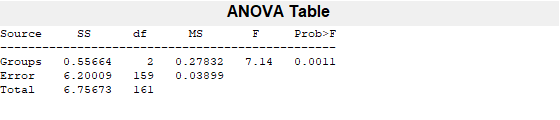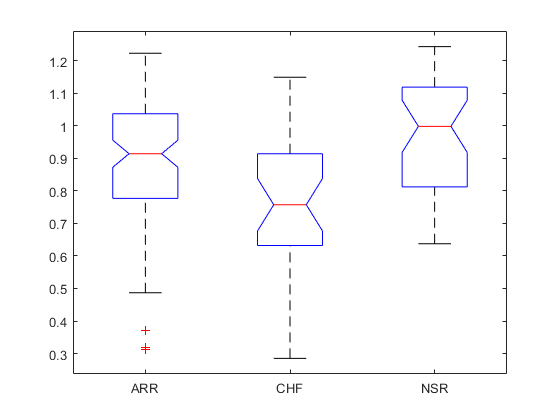As an additional example, consider the difference in variance in the second-lowest frequency (second-largest scale) wavelet subband for the three groups.

boxplot(allFeatures(:,featureindices.WVARfeatures(end-1)),allLabels,'notch','on') ylabel('Wavelet Variance') title('Wavelet Variance by Group') grid on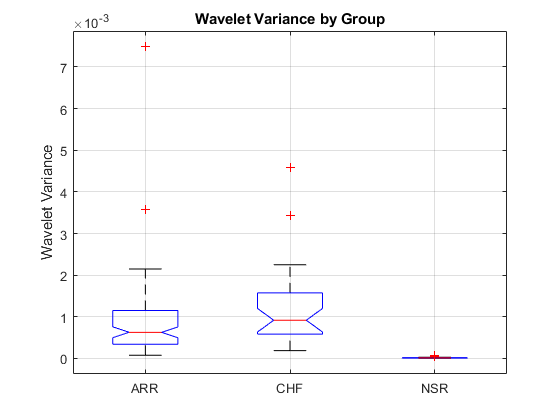If you perform an analysis of variance on this feature, you find that the NSR group has significantly lower variance in this wavelet subband than the ARR and CHF groups. These examples are just intended to illustrate how individual features serve to separate the classes. While one feature alone is not sufficient, the goal is to obtain a rich enough feature set to enable a classifier to separate all three classes.

### Signal Classification

Now that the data has been reduced to a feature vector for each signal, the next step is to use these feature vectors for classifying the ECG signals. You can use the Classification Learner app to quickly evaluate a large number of classifiers. In this example, a multi-class SVM with a quadratic kernel is used. Two analyses are performed. First we use the entire dataset (training and testing sets) and estimate the misclassification rate and confusion matrix using 5-fold cross-validation.

features = [trainFeatures; testFeatures]; rng(1) template = templateSVM(... 'KernelFunction','polynomial',... 'PolynomialOrder',2,... 'KernelScale','auto',... 'BoxConstraint',1,... 'Standardize',true); model = fitcecoc(... features,... [trainLabels;testLabels],... 'Learners',template,... 'Coding','onevsone',... 'ClassNames',{'ARR','CHF','NSR'}); kfoldmodel = crossval(model,'KFold',5); classLabels = kfoldPredict(kfoldmodel); loss = kfoldLoss(kfoldmodel)*100 [confmatCV,grouporder] = confusionmat([trainLabels;testLabels],classLabels); 
loss = 8.0247 

The 5-fold classification error is 8.02% (91.98% correct). The confusion matrix, confmatCV, shows which records were misclassified. grouporder gives the ordering of the groups. Two of the ARR group were misclassified as CHF, eight of the CHF group were misclassified as ARR and one as NSR, and two from the NSR group were misclassified as ARR.

### Precision, Recall, and F1 Score

In a classification task, the precision for a class is the number of correct positive results divided by the number of positive results. In other words, of all the records that the classifier assigns a given label, what proportion actually belong to the class. Recall is defined as the number of correct labels divided by the number of labels for a given class. Specifically, of all the records belonging to a class, what proportion did our classifier label as that class. In judging the accuracy your machine learning system, you ideally want to do well on both precision and recall. For example, suppose we had a classifier that labeled every single record as ARR. Then our recall for the ARR class would be 1 (100%). All records belonging to the ARR class would be labeled ARR. However, the precision would be low. Because our classifier labeled all records as ARR, there would be 66 false positives in this case for a precision of 96/162, or 0.5926. The F1 score is the harmonic mean of precision and recall and therefore provides a single metric that summarizes the classifier performance in terms of both recall and precision. The following helper function computes the precision, recall, and F1 scores for the three classes. You can see how helperPrecisionRecall computes precision, recall, and the F1 score based on the confusion matrix by examining the code in the Supporting Functions section.

CVTable = helperPrecisionRecall(confmatCV); 

You can display the table returned by helperPrecisionRecall with the following command.

disp(CVTable) 
 Precision Recall F1_Score _________ ______ ________ ARR 90.385 97.917 94 CHF 91.304 70 79.245 NSR 97.143 94.444 95.775 

Both precision and recall are good for the ARR and NSR classes, while recall is significantly lower for the CHF class.

For the next analysis, we fit a multi-class quadratic SVM to the training data only (70%) and then use that model to make predictions on the 30% of the data held out for testing. There are 49 data records in the test set.

model = fitcecoc(... trainFeatures,... trainLabels,... 'Learners',template,... 'Coding','onevsone',... 'ClassNames',{'ARR','CHF','NSR'}); predLabels = predict(model,testFeatures); 

Use the following to determine the number of correct predictions and obtain the confusion matrix.

correctPredictions = strcmp(predLabels,testLabels); testAccuracy = sum(correctPredictions)/length(testLabels)*100 [confmatTest,grouporder] = confusionmat(testLabels,predLabels); 
testAccuracy = 97.9592 

The classification accuracy on the test dataset is approximately 98% and the confusion matrix shows that one CHF record was misclassified as NSR.

Similar to what was done in the cross-validation analysis, obtain precision, recall, and the F1 scores for the test set.

testTable = helperPrecisionRecall(confmatTest); disp(testTable) 
 Precision Recall F1_Score _________ ______ ________ ARR 100 100 100 CHF 100 88.889 94.118 NSR 91.667 100 95.652 

### Classification on Raw Data and Clustering

Two natural questions arise from the previous analysis. Is feature extraction necessary in order to achieve good classification results? Is a classifier necessary, or can these features separate the groups without a classifier? To address the first question, repeat the cross-validation results for the raw time series data. Note that the following is a computationally expensive step because we are applying the SVM to a 162-by-65536 matrix. If you do not wish to run this step yourself, the results are described in the next paragraph.

rawData = [trainData;testData]; Labels = [trainLabels;testLabels]; rng(1) template = templateSVM(... 'KernelFunction','polynomial', ... 'PolynomialOrder',2, ... 'KernelScale','auto', ... 'BoxConstraint',1, ... 'Standardize',true); model = fitcecoc(... rawData,... [trainLabels;testLabels],... 'Learners',template,... 'Coding','onevsone',... 'ClassNames',{'ARR','CHF','NSR'}); kfoldmodel = crossval(model,'KFold',5); classLabels = kfoldPredict(kfoldmodel); loss = kfoldLoss(kfoldmodel)*100 [confmatCVraw,grouporder] = confusionmat([trainLabels;testLabels],classLabels); rawTable = helperPrecisionRecall(confmatCVraw); disp(rawTable) 
loss = 33.3333 Precision Recall F1_Score _________ ______ ________ ARR 64 100 78.049 CHF 100 13.333 23.529 NSR 100 22.222 36.364 

The misclassification rate for the raw time series data is 33.3%. Repeating the precision, recall, and F1 score analysis reveals very poor F1 scores for both the CHF (23.52) and NSR groups (36.36). Obtain the magnitude discrete Fourier transform (DFT) coefficients for each signal to perform the analysis in frequency domain. Because the data are real-valued, we can achieve some data reduction using the DFT by exploiting the fact that Fourier magnitudes are an even function.

rawDataDFT = abs(fft(rawData,[],2)); rawDataDFT = rawDataDFT(:,1:2^16/2+1); rng(1) template = templateSVM(... 'KernelFunction','polynomial',... 'PolynomialOrder',2,... 'KernelScale','auto',... 'BoxConstraint',1,... 'Standardize',true); model = fitcecoc(... rawDataDFT,... [trainLabels;testLabels],... 'Learners',template,... 'Coding','onevsone',... 'ClassNames',{'ARR','CHF','NSR'}); kfoldmodel = crossval(model,'KFold',5); classLabels = kfoldPredict(kfoldmodel); loss = kfoldLoss(kfoldmodel)*100 [confmatCVDFT,grouporder] = confusionmat([trainLabels;testLabels],classLabels); dftTable = helperPrecisionRecall(confmatCVDFT); disp(dftTable) 
loss = 19.1358 Precision Recall F1_Score _________ ______ ________ ARR 76.423 97.917 85.845 CHF 100 26.667 42.105 NSR 93.548 80.556 86.567 

Using the DFT magnitudes reduces the misclassification rate to 19.13% but that is still more than twice the error rate obtained with our 190 features. These analyses demonstrate that the classifier has benefited from a careful selection of features.

To answer the question concerning the role of the classifier, attempt to cluster the data using only the feature vectors. Use k-means clustering along with the gap statistic to determine both the optimal number of clusters and cluster assignment. Allow for the possibility of 1 to 6 clusters for the data.

rng default eva = evalclusters(features,'kmeans','gap','KList',[1:6]); eva 
eva = GapEvaluation with properties: NumObservations: 162 InspectedK: [1 2 3 4 5 6] CriterionValues: [1.2777 1.3539 1.3644 1.3570 1.3591 1.3752] OptimalK: 3 

The gap statistic indicates that the optimal number of clusters is three. However, if you look at the number of records in each of the three clusters, you see that the k-means clustering based on the feature vectors has done a poor job of separating the three diagnostic categories.

countcats(categorical(eva.OptimalY)) 
ans = 61 74 27 

Recall that there are 96 persons in the ARR class, 30 in the CHF class, and 36 in the NSR class.

### Summary

This example used signal processing to extract wavelet features from ECG signals and used those features to classify ECG signals into three classes. Not only did the feature extraction result in a significant amount of data reduction, it also captured the differences between the ARR, CHF, and NSR classes as demonstrated by the cross-validation results and the performance of the SVM classifier on the test set. The example further demonstrated that applying a SVM classifier to the raw data resulted in poor performance as did clustering the feature vectors without using a classifier. Neither the classifier nor the features alone were sufficient to separate the classes. However, when feature extraction was used as a data reduction step prior to the use of a classifier, the three classes were well separated.

### References

1. Baim DS, Colucci WS, Monrad ES, Smith HS, Wright RF, Lanoue A, Gauthier DF, Ransil BJ, Grossman W, Braunwald E. Survival of patients with severe congestive heart failure treated with oral milrinone. J American College of Cardiology 1986 Mar; 7(3):661-670.

2. Engin, M., 2004. ECG beat classification using neuro-fuzzy network. Pattern Recognition Letters, 25(15), pp.1715-1722.

3. Goldberger AL, Amaral LAN, Glass L, Hausdorff JM, Ivanov PCh, Mark RG, Mietus JE, Moody GB, Peng C-K, Stanley HE. PhysioBank, PhysioToolkit,and PhysioNet: Components of a New Research Resource for Complex Physiologic Signals. Circulation. Vol. 101, No. 23, 13 June 2000, pp. e215-e220. http://circ.ahajournals.org/content/101/23/e215.full

4. Leonarduzzi, R.F., Schlotthauer, G., and Torres. M.E. 2010. Wavelet leader based multifractal analysis of heart rate variability during myocardial ischaemia. Engineering in Medicine and Biology Society (EMBC), 2010 Annual International Conference of the IEEE.

5. Li, T. and Zhou, M., 2016. ECG classification using wavelet packet entropy and random forests. Entropy, 18(8), p.285.

6. Maharaj, E.A. and Alonso, A.M. 2014. Discriminant analysis of multivariate time series: Application to diagnosis based on ECG signals. Computational Statistics and Data Analysis, 70, pp. 67-87.

7. Moody GB, Mark RG. The impact of the MIT-BIH Arrhythmia Database. IEEE Eng in Med and Biol 20(3):45-50 (May-June 2001). (PMID: 11446209)

8. Zhao, Q. and Zhang, L., 2005. ECG feature extraction and classification using wavelet transform and support vector machines. IEEE International Conference on Neural Networks and Brain,2, pp. 1089-1092.

### Supporting Functions

helperPlotRandomRecords Plots four ECG signals randomly chosen from ECGData.

function helperPlotRandomRecords(ECGData,randomSeed) % This function is only intended to support the XpwWaveletMLExample. It may % change or be removed in a future release. if nargin==2 rng(randomSeed) end M = size(ECGData.Data,1); idxsel = randperm(M,4); for numplot = 1:4 subplot(2,2,numplot) plot(ECGData.Data(idxsel(numplot),1:3000)) ylabel('Volts') if numplot > 2 xlabel('Samples') end title(ECGData.Labels{idxsel(numplot)}) end end 

helperExtractFeatures Extracts the wavelet features and AR coefficients for blocks of the data of a specified size. The features are concatenated into feature vectors.

function [trainFeatures, testFeatures,featureindices] = helperExtractFeatures(trainData,testData,T,AR_order,level) % This function is only in support of XpwWaveletMLExample. It may change or % be removed in a future release. trainFeatures = []; testFeatures = []; for idx =1:size(trainData,1) x = trainData(idx,:); x = detrend(x,0); arcoefs = blockAR(x,AR_order,T); se = shannonEntropy(x,T,level); [cp,rh] = leaders(x,T); wvar = modwtvar(modwt(x,'db2'),'db2'); trainFeatures = [trainFeatures; arcoefs se cp rh wvar']; %#ok<AGROW> end for idx =1:size(testData,1) x1 = testData(idx,:); x1 = detrend(x1,0); arcoefs = blockAR(x1,AR_order,T); se = shannonEntropy(x1,T,level); [cp,rh] = leaders(x1,T); wvar = modwtvar(modwt(x1,'db2'),'db2'); testFeatures = [testFeatures;arcoefs se cp rh wvar']; %#ok<AGROW> end featureindices = struct(); % 4*8 featureindices.ARfeatures = 1:32; startidx = 33; endidx = 33+(16*8)-1; featureindices.SEfeatures = startidx:endidx; startidx = endidx+1; endidx = startidx+7; featureindices.CP2features = startidx:endidx; startidx = endidx+1; endidx = startidx+7; featureindices.HRfeatures = startidx:endidx; startidx = endidx+1; endidx = startidx+13; featureindices.WVARfeatures = startidx:endidx; end function se = shannonEntropy(x,numbuffer,level) numwindows = numel(x)/numbuffer; y = buffer(x,numbuffer); se = zeros(2^level,size(y,2)); for kk = 1:size(y,2) wpt = modwpt(y(:,kk),level); % Sum across time E = sum(wpt.^2,2); Pij = wpt.^2./E; % The following is eps(1) se(:,kk) = -sum(Pij.*log(Pij+eps),2); end se = reshape(se,2^level*numwindows,1); se = se'; end function arcfs = blockAR(x,order,numbuffer) numwindows = numel(x)/numbuffer; y = buffer(x,numbuffer); arcfs = zeros(order,size(y,2)); for kk = 1:size(y,2) artmp = arburg(y(:,kk),order); arcfs(:,kk) = artmp(2:end); end arcfs = reshape(arcfs,order*numwindows,1); arcfs = arcfs'; end function [cp,rh] = leaders(x,numbuffer) y = buffer(x,numbuffer); cp = zeros(1,size(y,2)); rh = zeros(1,size(y,2)); for kk = 1:size(y,2) [~,h,cptmp] = dwtleader(y(:,kk)); cp(kk) = cptmp(2); rh(kk) = range(h); end end 

helperPrecisionRecall returns the precision, recall, and F1 scores based on the confusion matrix. Outputs the results as a MATLAB table.

function PRTable = helperPrecisionRecall(confmat) % This function is only in support of XpwWaveletMLExample. It may change or % be removed in a future release. precisionARR = confmat(1,1)/sum(confmat(:,1))*100; precisionCHF = confmat(2,2)/sum(confmat(:,2))*100 ; precisionNSR = confmat(3,3)/sum(confmat(:,3))*100 ; recallARR = confmat(1,1)/sum(confmat(1,:))*100; recallCHF = confmat(2,2)/sum(confmat(2,:))*100; recallNSR = confmat(3,3)/sum(confmat(3,:))*100; F1ARR = 2*precisionARR*recallARR/(precisionARR+recallARR); F1CHF = 2*precisionCHF*recallCHF/(precisionCHF+recallCHF); F1NSR = 2*precisionNSR*recallNSR/(precisionNSR+recallNSR); % Construct a MATLAB Table to display the results. PRTable = array2table([precisionARR recallARR F1ARR;... precisionCHF recallCHF F1CHF; precisionNSR recallNSR... F1NSR],'VariableNames',{'Precision','Recall','F1_Score'},'RowNames',... {'ARR','CHF','NSR'}); end 

## SupportGet trial now